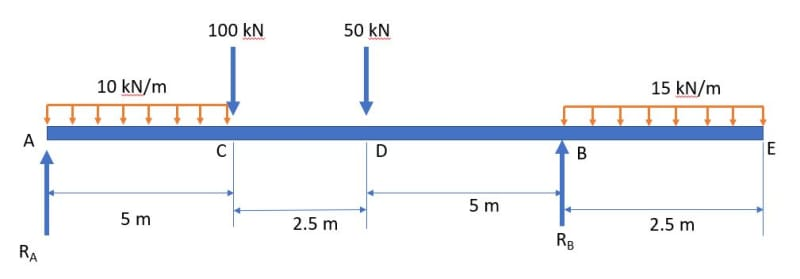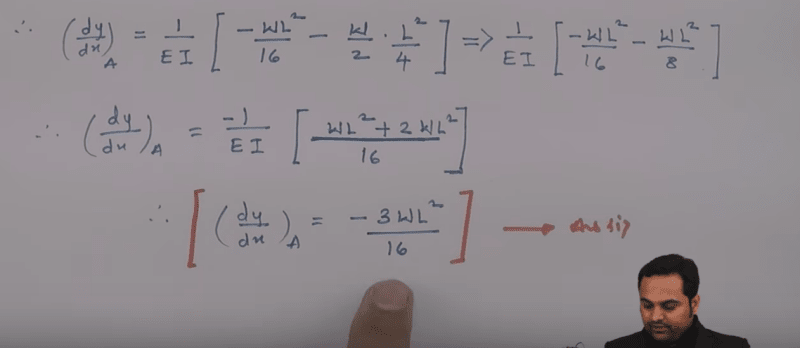×
INTELLIGENT WORK FORUMS
FOR ENGINEERING PROFESSIONALS

Are you an
Engineering professional?
Join Eng-Tips Forums!
• Talk With Other Members
• Be Notified Of Responses
• Keyword Search
Favorite Forums
• Automated Signatures
• Best Of All, It's Free!

*Eng-Tips's functionality depends on members receiving e-mail. By joining you are opting in to receive e-mail.

Posting Guidelines

Promoting, selling, recruiting, coursework and thesis posting is forbidden.

Beam deflection problem with multiple Uniformly Distributed Loads

Beam deflection problem with multiple Uniformly Distributed Loads

(OP)Hi all,

I am trying to solve this beam problem to find the deflection at different points along the length of the beam. However I am having a hard time writing the equation for bending moment using Macaulay's theorem since there are 2 uniformly distributed loads of different magnitudes.

Can someone help solve this problem?

RE: Beam deflection problem with multiple Uniformly Distributed Loads

Your structure is statically determinate, so you can solve for RA and RB directly. From there, you can find the moment at any point distant 'x' from Point A using simple statics.

Macaulay's Theorem is just another name for the Double Integration Method. I was going to refer you to the following for a simple example of a beam with a point load at midspan. Unfortunately, the man in the video gets the expression for dy/dx (slope) and y (deflection) wrong. Can you find his error?

Personally, I would not use the Macaulay Theorem to solve this problem, unless you are required to use it in your assignment. There is nothing wrong with the method, but with that many loads, it is very easy to drop a term or make some arithmetic error. There are a number of easier ways to solve it. One method would be to treat each load separately, then use superposition to find the combined answer for slope and deflection at any point distant 'x' from Point A.

We can't solve your problem for you, but it really is pretty straightforward, even though it has a lot of terms. Start by solving for the two reactions, then express the moment at any point distant 'x' from Point A and show us what you've got.

BA

RE: Beam deflection problem with multiple Uniformly Distributed Loads

There is not a one-equation continuous solution. There are four (4) equations needed. One of the 4 equations will be valid within each range between loading conditions.

RE: Beam deflection problem with multiple Uniformly Distributed Loads

Quote (1503-44)

There is not a one-equation continuous solution. There are four (4) equations needed. One of the 4 equations will be valid within each range between loading conditions.
Correct, although there are similarities in the equations, some having additional terms accounting for different ranges. That point was missed by the instructor in the video which I mentioned earlier. He used the wrong expression when finding the slope at Point A and came up with the wrong answer. (see below)Although he came up with the correct answer for deflection at midspan, his expression for deflection was not valid for the left half of the beam.

BA

RE: Beam deflection problem with multiple Uniformly Distributed Loads

If boolean expressions are used, a single equation can be written for the whole span.

Mx = RA.x - P(x-a)(x>a) - Q(x-b)(x>b) - R(x-c)(x>c)

is the moment at any point of a simple span beam, distant x from Point A, with point loads P, Q and R at a, b and c respectively from Point A. The boolean expressions, shown in red have the value of 1 or 0 depending on whether the bracketed expression is true or false.

BA

RE: Beam deflection problem with multiple Uniformly Distributed Loads

(OP)
I was confusing myself thinking I could write one nice complete moment equation that would tell me the deflection at any point. but that is wrong because the bending behavior differs at each section of the beam.

I was actually able to write moment equations for individual sections and integrated them twice(which ended up with a lot of constants of integration - 8 in total). Then I applied multiple boundary conditions to solve for all the constants. boundary conditions were:
1. deflection = 0 at supports - (solved for 3 constants).
2. deflection is the same at a particular point, meaning I could equate the deflections given by 2 different equations (points C and D) - (solved for 2 constants).
3. slope is the same at a particular point, meaning I could equate the slopes given by 2 different equations (points C, D and B) - (solved for 3 constants).

Thus I now have different bending moment, slope and deflection equations for different sections of the beams!

thank you all for the great inputs :)

RE: Beam deflection problem with multiple Uniformly Distributed Loads

Happy to hear you found the solution. Congrats!

BA

RE: Beam deflection problem with multiple Uniformly Distributed Loads

BAretired,

I was taught to write equations like that as...

Mx = RAx - P<x-a> - Q<x-b> - R<x-c>,

with the pointy brackets being zero if the contained value was equal to or less than.

--
JHG

RE: Beam deflection problem with multiple Uniformly Distributed Loads

Okay, that is clear and easier to write. I have not run into that notation until now, but it makes sense.

BA

RE: Beam deflection problem with multiple Uniformly Distributed Loads

Great. you found your solution. happy to see this.

Red Flag This Post

Please let us know here why this post is inappropriate. Reasons such as off-topic, duplicates, flames, illegal, vulgar, or students posting their homework.

Red Flag Submitted

Thank you for helping keep Eng-Tips Forums free from inappropriate posts.
The Eng-Tips staff will check this out and take appropriate action.

Resources

Low-Volume Rapid Injection Molding With 3D Printed Molds
Learn methods and guidelines for using stereolithography (SLA) 3D printed molds in the injection molding process to lower costs and lead time. Discover how this hybrid manufacturing process enables on-demand mold fabrication to quickly produce small batches of thermoplastic parts. Download Now
Examine how the principles of DfAM upend many of the long-standing rules around manufacturability - allowing engineers and designers to place a partâ€™s function at the center of their design considerations. Download Now
Industry Perspective: Education and Metal 3D Printing
Metal 3D printing has rapidly emerged as a key technology in modern design and manufacturing, so itâ€™s critical educational institutions include it in their curricula to avoid leaving students at a disadvantage as they enter the workforce. Download Now

Close Box

Join Eng-Tips® Today!

Join your peers on the Internet's largest technical engineering professional community.
It's easy to join and it's free.

Here's Why Members Love Eng-Tips Forums:

•Talk To Other Members
• Notification Of Responses To Questions
• Favorite Forums One Click Access
• Keyword Search Of All Posts, And More...

Register now while it's still free!